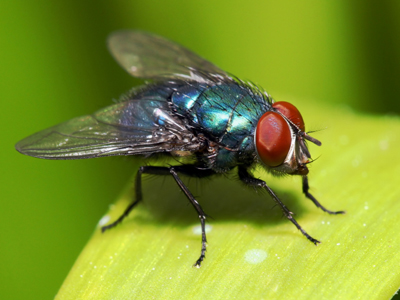You would use millimetres to measure a fly.

# Measures (Year 4)

As children progress through KS2 they will learn more about measures in Maths. As well as understanding the different values of the metric measures (for example that 2 litres = 2,000 millilitres), in Year Four children will be exposed to some of the old imperial measures such as pints. They will also learn how to write measurements using more than one type of measure, for example 2.5 metres as 2m 50cm.

To measure liquid capacity, millilitres, centilitres and litres are used. These are called metric measures but we some times use other measures like gallons or pints. These are known as imperial measures. There are more imperial measures that we sometimes use - miles for long distances, stones for weights and inches for short distances instead of the metric kilometres, kilograms and centimetres.

Do you know your litres from your pints? Test your knowledge of measures by playing this quiz.

1.
What is one tenth of a litre in millilitres?
0.1ml
1ml
10ml
100ml
There are 1,000 ml in a litre so divide by 10
2.
How many grams are equivalent to one quarter kg?
25g
250g
250.5g
2,500g
There are 1,000g in a kg so divide by 4
3.
10 millimetres is equal to?
1cm
10cm
100cm
1,000cm
1m = 100cm = 1,000mm
4.
Which of these statements is true?
Pint > 1 litre
Pint = 1 litre
Pint > 0.5 litre
Pint = 2 litres
A pint is slightly more than half a litre
5.
What is half of 1 metre in cm?
0.5cm
5cm
50cm
500cm
1m = 100cm so a half of 100 is 50
6.
How do we write 451cm in m?
0.451m
4.51m
45.1m
451m
Divide by 100 to convert centimetres to metres
7.
How many millimetres in one metre?
10mm
100mm
1,000mm
10,000mm
The prefix 'milli' means the same as 1?1,000
8.
Which of the following could be the weight of a small puppy, for instance a Chihuahua?
100g
10kg
100kg
50g
A larger puppy, such as a Great Dane, can weigh as much as 1kg at birth!
9.
Which unit would you use to measure the length of a fly?
Kilometre
Millimetre
Centimetre
Metre
Flies are very small so mm would be best
10.
What is 6.45m expressed in metres and cm?
6m 45cm
64m 5cm
0m 645cm
645m 0cm
Here the numbers to the left of the decimal point are metres. The first two numbers to the right of the decimal point are cm. If there was a third number to the right of the decimal point then all three would be mm